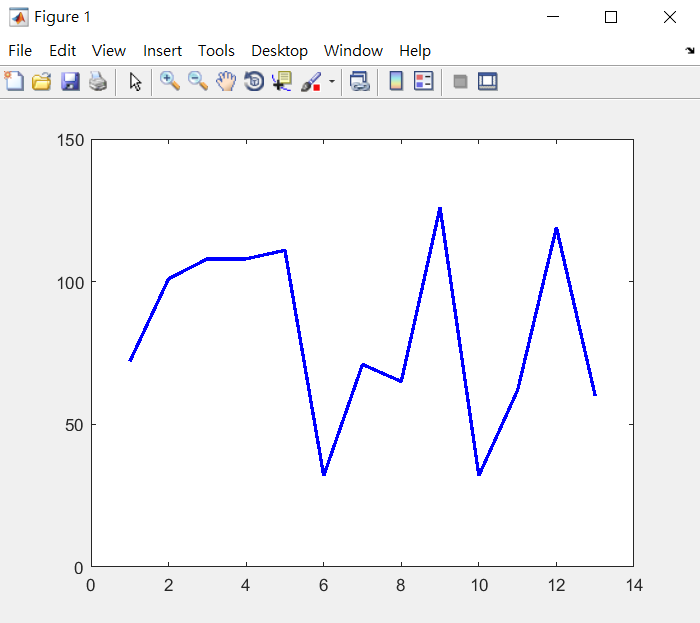### 【MATLAB】應用基因演算法(二)：找尋正確字串

• 1321
• 0
• GA
• 2018-12-26

【MATLAB】應用基因演算法(一)：Peaks 函數求極值

## 'Hello GA~ >w<'

TargStr = 'Hello GA~ >w<';

>> plot(double(TargStr), 'LineWidth', 2, 'Color', 'b')## 1. 變數數量

VarNumber = numel(TargStr);

## 2. 上下邊界圖片來源：Excel-製作ASCII碼對照表

% 上下邊界設定
% 注意：空白鍵的代碼是 32 ，所以下邊界從 32 開始。
lb = zeros(1, VarNumber)+32;
ub = zeros(1, VarNumber)+127;

## 3. 目標函數

function scores = FitnessFcn(x, TargStr)
%% 適應函數

% 把目標字串轉換成 double 一維向量
TS = double(TargStr);

% GA 染色體與目標字串相減取絕對值，作為評分標準。
% 其分數愈接近 0 ，表示愈接近目標字串。
scores = sum(abs(x-TS));

end

fitfcn = @(x) FitnessFcn(x, TargStr);

scores = sum( x == TS );


## 4. 繪圖函數

function state = myPlot(options, state, flag, TargStr)
%% 自定繪圖函數，畫出每次迭代的結果。

% 叫出目前最佳的適應函數值
[~, i] = min(state.Score);
X = state.Population(i, :);

% 清空上一張圖
cla

% 繪製目標函數圖形
plot(double(TargStr), 'Color', 'b', 'LineWidth', 2)

% 把目前適應函數值，疊到同一張圖面上
hold on
plot(X, 'Color', 'r', 'LineWidth', 2, 'LineStyle', '--')

% 於標題展示目前最佳化結果
title(['Tar:', TargStr, '  /  Pred:', cellfun(@char, num2cell(X))])

% 坐標軸設定
axis([0, numel(TargStr)+1, 0, 150])

% 即時顯示
drawnow

end

my_plot = @(options, state, flag) myPlot(options, state, flag, TargStr);

## 5. GA 選項設定

options = optimoptions('ga', ...                       % 最佳化算法
'PopulationSize', 50, ...       % 染色體數量
'MaxGenerations', 500, ...      % 最大繁衍代數
'MaxStallGenerations', 100, ... % 最大停滯代數
'PlotFcn', my_plot, ...         % 自定義繪圖函數
'Display', 'iter');   

## 6. 執行 GA

x = ga(fitfcn, VarNumber, [], [], [], [], lb, ub, [], 1:VarNumber, options);

以下為程式執行結果：

## 小結

### 現在有五種貨品要準備上貨櫃，貨櫃內有 20 位置，又每個貨品大小重量皆不同。 請問該怎麼裝哪些貨物，才能達到最大載重？

【MATLAB】應用基因演算法(三)：高效率的送貨員

## 附錄

%% GA 範例程式
% 功能：找目標字串
%
% 2018.07.08, Shayne.

TargStr = 'Hello GA~ >w<';
VarNumber = numel(TargStr);

lb = zeros(1, VarNumber)+32;
ub = zeros(1, VarNumber)+127;

fitfcn = @(x) FitnessFcn(x, TargStr);
my_plot = @(options, state, flag) myPlot(options, state, flag, TargStr);

options = optimoptions('ga', ...
'PopulationSize', 50, ...
'MaxGenerations', 500, ...
'MaxStallGenerations', 100, ...
'PlotFcn', my_plot, ...
'Display', 'iter');

x = ga(fitfcn, VarNumber, [], [], [], [], lb, ub, [], 1:VarNumber, options);

function scores = FitnessFcn(x, TargStr)
TS = double(TargStr);
scores = sum(abs(x-TS));
end

function state = myPlot(options, state, flag, TargStr)
[~, i] = min(state.Score);
X = state.Population(i, :);

cla
plot(double(TargStr), 'Color', 'b', 'LineWidth', 2)

hold on
plot(X, 'Color', 'r', 'LineWidth', 2, 'LineStyle', '--')

title(['Tar:', TargStr, '  /  Pred:', cellfun(@char, num2cell(X))])
axis([0, numel(TargStr)+1, 0, 150])
drawnow
end